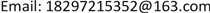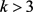﻿ 利用取整函数解决啤酒瓶换啤酒问题 Using Integer Function to Solve the Problem of Replacing Beer Bottle for Beer

Pure Mathematics
Vol. 09  No. 06 ( 2019 ), Article ID: 31577 , 7 pages
10.12677/PM.2019.96090

Using Integer Function to Solve the Problem of Replacing Beer Bottle for Beer

Shujing Li, Nannan Liu

School of Mathematics and Statistics, Qinghai University for Nationalities, Xining QinghaiReceived: Jul. 9th, 2019; accepted: Jul. 19th, 2019; published: Aug. 5th, 2019ABSTRACT

The rounding function is a common function. Its form is simple, its nature is very unique, and it is widely used in the problems of seeking limits, seeking and integrating. Applying the nature of the rounding function, a mapping of the real number set of the beer bottle to the beer to a set of integers is established, and any real number is converted into an integer to solve how to optimize the beer. By transforming the problem of beer bottle change for beer in supermarket promotion into a mathematical model, some results are derived according to the nature of the rounding function; and the mathematical model is deeply explored and extended theoretically, and a general conclusion is obtained. Combined with the conclusions we have obtained, the conclusions are further applied to simplify the actual problems.

Keywords:Beer Bottles for Beer, Rounding Function, Limit, Profit1. 引言

2. 预备知识

$⌊x⌋\le x$$⌈x⌉\ge x$$⌊x⌋=x$ $⇔$ x是一个整数 $⇔⌈x⌉=x$

$\begin{array}{l}⌊x⌋-⌈x⌉=1;\\ x-1<⌊x⌋\le ⌈x⌉

$\begin{array}{l}⌊x⌋=n⇔n\le x

$\begin{array}{l}x

$⌊f\left(x\right)⌋=⌊f\left(⌊x⌋\right)⌋$

$⌈f\left(x\right)⌉=⌈f\left(⌈x⌉\right)⌉$

$\begin{array}{l}⌊\sqrt{⌊x⌋}⌋=⌊x⌋;\\ \sqrt{⌈x⌉}=⌈\sqrt{x}⌉\end{array}$

3. 问题的提出

1) 易知，一次购买10瓶时，按“4个啤酒瓶换1瓶”的规定，可换3瓶啤酒。如果一次购买20瓶时，可换多少瓶啤酒？会是6瓶吗？

2) 当一次购买的数量很多时，比如，1000瓶、99,999瓶，如何算出可换多少瓶啤酒？

3) 假设每瓶啤酒的价格是a元，则花10a元可得12瓶，平均每瓶合10a/12元，小于a元/瓶。

4) 从理论上讲，当一次购买的数量为无穷大时，可换啤酒量占购买量的比会是一个常数吗？

5) 从理论上讲，当一次购买的数量为无穷大时，按照规定，商家会赔吗？平均每瓶啤酒的价格会降到0吗？如不是，会是多少？

6) 假设现有900人，每人只喝3瓶啤酒，按照规定，请问一次购买多少瓶即可满足每人3瓶？

4. 问题的探究

1) 数学模型的建立

$⌊1+\frac{n-4}{3}⌋=⌊\frac{n-1}{3}⌋$

2) 当购买很多啤酒时，换得的啤酒数量ΔN占购买量n多少？

$n\to \infty$ 时， $\frac{\Delta N}{n}$ 是否有极限？若有，极限是多少？

$\frac{1}{n}\left(\frac{n-1}{3}-1\right)<\frac{1}{n}⌊\frac{n-1}{3}⌋\le \frac{1}{n}\frac{n-1}{3}$

$\frac{1}{n}\left(\frac{n-4}{3}\right)<\frac{1}{n}⌊\frac{n-1}{3}⌋\le \frac{1}{n}\frac{n-1}{3}$

$\underset{n\to \infty }{\text{lim}}\frac{1}{n}\frac{n-4}{3}=\underset{n\to \infty }{\mathrm{lim}}\frac{1}{n}\frac{n-1}{3}=\frac{1}{3}$

$\underset{n\to \infty }{\text{lim}}\frac{\Delta N}{n}=\frac{1}{3}$

$\frac{\Delta N}{n}=0.333333296$

$\Delta N=⌊\frac{10000000000-1}{3}⌋=⌊3333333333⌋=3333333333$

$\frac{\Delta N}{n}=0.333333333$

3) 由简单到复杂

$\underset{n\to \infty }{\mathrm{lim}}\frac{1}{n}\frac{n-k}{k-1}=\underset{n\to \infty }{\mathrm{lim}}\frac{1}{n}\frac{n-1}{k-1}=\frac{1}{k-1}$

4) 设k个空瓶换m瓶( $k>m$$k\in N$$m\in N$ 常数)。

$\frac{1}{n}\frac{mn-mm-k+m}{k-m}=\frac{1}{n}\left(\frac{mn-mm}{k-m}-1\right)<\frac{1}{n}\cdot ⌊\frac{mn-mm}{k-m}⌋\le \frac{1}{n}\cdot \frac{mn-mm}{k-m}$

$\underset{n\to \infty }{\text{lim}}\frac{1}{n}\frac{mn-mm}{k-m}=\frac{m}{k-m}$

$\underset{n\to \infty }{\mathrm{lim}}\frac{na}{n+\Delta N}=\underset{n\to \infty }{\mathrm{lim}}\frac{na}{n+⌊\frac{mn-mm}{k-m}⌋}=\underset{n\to \infty }{\mathrm{lim}}\frac{na}{\frac{nk-mm}{k-m}}=\frac{k-m}{k}a$

$\underset{n\to \infty }{\mathrm{lim}}\frac{n\left(a-b\right)}{n+\Delta N}=\underset{n\to \infty }{\mathrm{lim}}\frac{n\left(a-b\right)}{n+⌊\frac{mn-mm}{k-m}⌋}=\underset{n\to \infty }{\mathrm{lim}}\frac{n\left(a-b\right)}{\frac{nk-mm}{k-m}}=\frac{k-m}{k}\left(a-b\right)$

5. 几类应用

$\begin{array}{l}2700\le ⌊\frac{4n-1}{3}⌋\\ n\ge \left[2025.25\right]=2026\end{array}$

$y=n\cdot \left(4-2\right)=2026×2=4052$

$z=n\cdot \left(\frac{4-1}{4}\right)\cdot 4=6078$

$3M\le \left[n+\Delta N\right]=n+⌊\frac{n-1}{3}⌋=⌊\frac{4n-1}{3}⌋$

$3M\le \frac{4n-1}{3}$

$n\ge \frac{9M+1}{4}$

$y=n\cdot \left(4-2\right)\ge \left(\frac{9M+1}{2}\right)\left(4-2\right)=\frac{9M+1}{2}$

$\text{z}=n\cdot \left(\frac{4-1}{4}\right)\cdot 4\ge \frac{9M+1}{4}×3=\frac{27M+3}{4}$

$3A\le \frac{nk-mm}{k-m}$ 从而

$n\ge \frac{3A\left(k-1\right)}{k}$

$y=n\cdot \left(4-2\right)\ge \frac{3A\left(k-1\right)}{k}\cdot 2=\frac{6A\left(k-1\right)}{k}$

$z=n\cdot \left(\frac{k-1}{k}\right)\cdot 4\ge \frac{3A\left(k-1\right)}{k}\cdot \frac{k-1}{k}=\frac{12A{\left(k-1\right)}^{2}}{{k}^{2}}$

$B\le \frac{nk-{m}^{2}}{k-m}$ ，从而

$n\ge \frac{B\left(k-m\right)+{m}^{2}}{k}$

$y=n\cdot \left(4-2\right)=\frac{2B\left(k-m\right)+2{m}^{2}}{k}$

$z=4n\cdot \frac{k-m}{k}\ge \frac{4B{\left(k-m\right)}^{2}+4\left(k-m\right){k}^{2}}{{k}^{2}}$

Using Integer Function to Solve the Problem of Replacing Beer Bottle for Beer[J]. 理论数学, 2019, 09(06): 679-685. https://doi.org/10.12677/PM.2019.96090

1. 1. 郑克明. 数论基础[M]. 重庆: 西南师范大学出版社, 1991.

2. 2. 陈思禄, 延伟, 李智明. 一类上取整函数的极小值问题[J]. 数学的实践与认识, 2015, 45(20): 299-304.

3. 3. 王玉霞. 关于函数y = [x]及其应用[J]. 高等数学研究, 2005, 8(4): 46-49.

4. 4. 詹玉. 极限在商业活动中的应用[J]. 荆楚理工学院学报(自然科学版), 2007, 22(6): 81-83.

5. 5. 詹玉. 取整函数在促销中的应用[J]. 宁波职业技术学院学报, 2008, 12(2): 15-17.